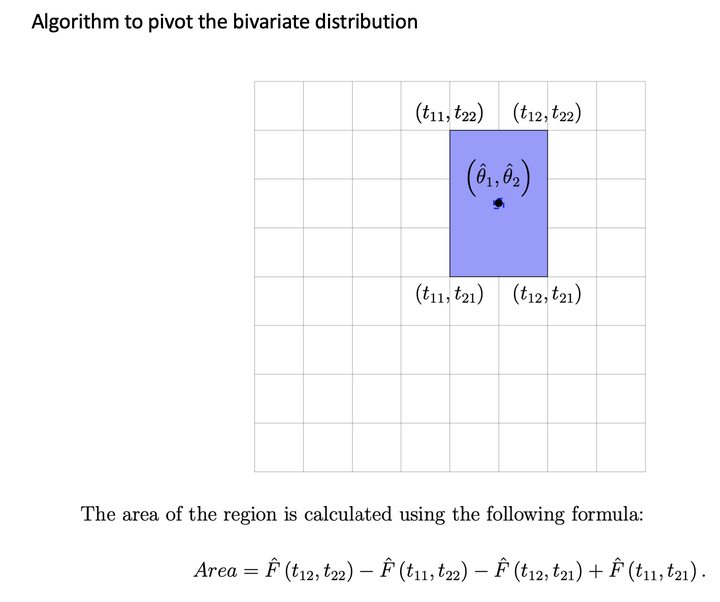# Multivariate Saddlepoint-Based Bootstrap Method (MSPBB)Wang (1990) devised an analytical approximation for the bivariate CDF for a rectangular set using the Lugannani and Rice (1980) formula and the standard bivariate normal distribution. We considered the theoretical study of the saddlepoint approximation for the bivariate distributions and provided necessary corrections and clarifications for the formula established by Wang (1990).

The numerical methods are used to compute the probability when the set is non-rectangular. There are many applications where inferences on a particular parameter $$\theta_{1}$$ in the presence of other $$k-1$$ parameters are necessary. Obtaining a marginal probability distribution for $$\hat{\theta}_{1}$$ is a straightforward matter of letting the arguments corresponding to the remaining parameters approach infinite values in the joint CDF of $$k$$ estimators. In order to approximate the joint CDF of estimators, we consider a class of problems in which the estimators $$\left[\hat{\theta}_{1}, \ldots, \hat{\theta}_{k}\right]^{T}$$ may be determined as the roots of $$k$$ distinct estimating equations.

## Application

We can construct a joint confidence region for multi-dimensional parameters.

## Method

First, we derive estimating equations as a vector of quadratic forms in normal random variables. Then, we identify the moment-generating function of quadratic forms in a normal random variable. Next, we derive the necessary and sufficient conditions to approximate the CDF of estimators via estimating equations. Finally, we invert the CDF of estimators to construct a joint confidence region.

## Software

• PhD dissertation Chapter I, section 1.2 supplement.

## Paper

• PhD dissertation Chapter I, section 1.2.